# Find maximum values & position in columns and rows of a Dataframe in Pandas

In this article, we are going to discuss how to find maximum value and its index position in columns and rows of a Dataframe.

### DataFrame.max()

Pandas dataframe.max() method finds the maximum of the values in the object and returns it. If the input is a series, the method will return a scalar which will be the maximum of the values in the series. If the input is a dataframe, then the method will return a series with maximum of values over the specified axis in the dataframe. The index axis is the default axis taken by this method.

Syntax : DataFrame.max(axis=None, skipna=None, level=None, numeric_only=None, **kwargs)

Parameters :
axis : {index (0), columns (1)}
skipna : Exclude NA/null values when computing the result
level : If the axis is a MultiIndex (hierarchical), count along a particular level, collapsing into a Series
numeric_only : Include only float, int, boolean columns. If None, will attempt to use everything, then use only numeric data. Not implemented for Series.

Returns : max : Series or DataFrame (if level specified)

Let’s take an example of how to use this function. Let’s suppose we have a Dataframe

 `import` `numpy as np ` `import` `pandas as pd ` `# List of Tuples ` `matrix ``=` `[(``10``, ``56``, ``17``), ` `          ``(np.NaN, ``23``, ``11``), ` `          ``(``49``, ``36``, ``55``), ` `          ``(``75``, np.NaN, ``34``), ` `          ``(``89``, ``21``, ``44``) ` `          ``] ` `# Create a DataFrame ` `abc ``=` `pd.DataFrame(matrix, index ``=` `list``(``'abcde'``), columns ``=` `list``(``'xyz'``)) ` ` `  `# output  ` `abc `

Output: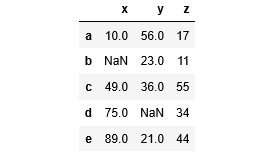### How to find Maximum values of every column?

To find the maximum value of each column, call `max()` method on the Dataframe object without taking any argument.

 `# find the maximum of each column ` `maxValues ``=` `abc.``max``() ` ` `  `print``(maxValues) `

Output :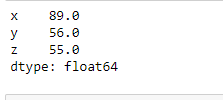We can see that it returned a series of maximum values where the index is column name and values are the maxima from each column.

### How to find maximum values of every row?

To find the maximum value of each row, call `max()` method on the Dataframe object with an argument axis = 1.

 `# find the maximum values of each row ` `maxValues ``=` `abc.``max``(axis ``=` `1``) ` `print``(maxValues) `

Output :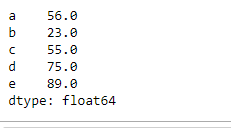We can see that it returned a series of maximum values where the index is row name and values are the maxima from each row. We can see that in the above examples NaN values are skipped while finding the maximum values in any axis. We can include NaN values as well if we want.

### How to find maximum values of every column without skipping NaN?

 `# find maximum value of each  ` `# column without skipping NaN ` `maxValues ``=` `abc.``max``(skipna ``=` `False``) ` ` `  `print``(maxValues) `

Output :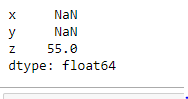By putting skipna=False we can include NaN values also. If any NaN value exists it will be considered as the maximum value.

### How to find maximum values of a single column or selected columns?

To get the maximum value of a single column see the following example

 `# find maximum value of a  ` `# single column 'x' ` `maxClm ``=` `df[``'x'``].``max``() ` ` `  `print``(``"Maximum value in column 'x': "` `)  ` `print``(maxClm) `

Output :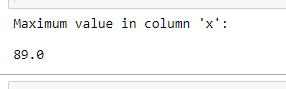We have another way to find maximum value of a column :

 `# find maximum value of a  ` `# single column 'x' ` `maxClm ``=` `df.``max``()[``'x'``] `

The result will be same as above.
Output:A list of columns can also be passed instead of a single column to find the maximum values of specified columns

 `# find maximum values of a list of columns ` `maxValues ``=` `df[[``'x'``, ``'z'``]].``max``() ` ` `  `print``(``"Maximum value in column 'x' & 'z': "``) ` `print``(maxValues) `

Output :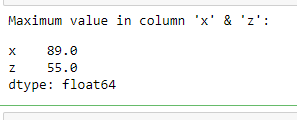### How to get position of maximum values of every column?

DataFrame.idxmax(): Pandas `dataframe.idxmax()` method returns index of first occurrence of maximum over requested axis. While finding the index of the maximum value across any index, all NA/null values are excluded.

Syntax: DataFrame.idxmax(axis=0, skipna=True)

Parameters :
axis : 0 or ‘index’ for row-wise, 1 or ‘columns’ for column-wise
skipna : Exclude NA/null values. If an entire row/column is NA, the result will be NA

Returns : idxmax : Series

Let’s take some examples to understand how to use it :

How to get row index label of Maximum value in every column

 `# find the index position of maximun ` `# values in every column ` `maxValueIndex ``=` `df.idxmax() ` ` `  `print``(``"Maximum values of columns are at row index position :"``) ` `print``(maxValueIndex) `

Output :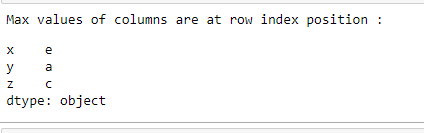It returns a series containing the column names as index and row as index labels where the maximum value exists in that column.

### How to find Column names of Maximum value in every row?

 `# find the column name of maximum ` `# values in every row ` `maxValueIndex ``=` `df.idxmax(axis ``=` `1``) ` ` `  `print``(``"Max values of row are at following columns :"``) ` `print``(maxValueIndex) `

Output :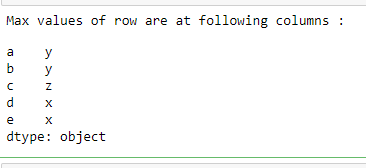It returns a series containing the rows index labels as index and column names as values where the maximum value exists in that row.

My Personal Notes arrow_drop_upCheck out this Author's contributed articles.

If you like GeeksforGeeks and would like to contribute, you can also write an article using contribute.geeksforgeeks.org or mail your article to contribute@geeksforgeeks.org. See your article appearing on the GeeksforGeeks main page and help other Geeks.

Please Improve this article if you find anything incorrect by clicking on the "Improve Article" button below.

Article Tags :

Be the First to upvote.

Please write to us at contribute@geeksforgeeks.org to report any issue with the above content.